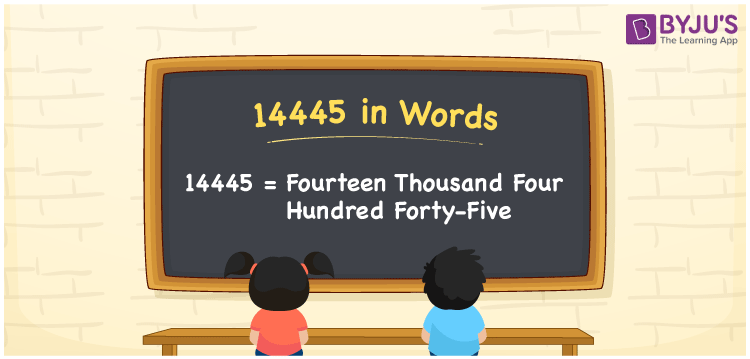# 14445 in Words

14445 in words is written as Fourteen thousand four hundred forty-five. In both the International System of Numerals and the Indian System of Numerals, 14445 is written as Fourteen thousand four hundred forty-five. The number 14445 is a Cardinal Number as it denotes some quantity. For example, “that mobile phone costs 14445 rupees”.

 14445 in Words Fourteen thousand four hundred forty-five Fourteen thousand four hundred forty-five in Number 14445

## 14445 in English Words

14445 in English words is read as “Fourteen thousand four hundred forty-five”.## How to Write 14445 in Words?

To write 14445 in words, we shall use the place value chart. In the place value chart, put 1 in the ten thousands, 4 in the thousands, hundreds, and tens and 5 in the ones. Let us make a place value chart to write the number 14445 in words.

 Ten Thousands Thousands Hundreds Tens Ones 1 4 4 4 5

Thus, we can write the expanded form as

1 × Ten Thousand + 4 × Thousand + 4 × Hundred + 4 × Ten + 5 × One

= 1 × 10000 + 4 × 1000 + 4 × 100 + 4 × 10 + 5 × 1

= 10000 + 4000 + 400 + 40 + 5

= 14445

= Fourteen thousand four hundred forty-five.

14445 is a natural number, the successor of 14444 and the predecessor of 14446.

14445 in words – Fourteen thousand four hundred forty-five

• Is 14445 an odd number? – Yes
• Is 14445 an even number? – No
• Is 14445 a perfect square number? – No
• Is 14445 a perfect cube number? – No
• Is 14445 a prime number? – No
• Is 14445 a composite number? – Yes

## Frequently Asked Questions on 14445 in Words

Q1

### How to write 14445 in words?

14445 in words is written as Fourteen thousand four hundred forty-five.
Q2

### How to write 14445 in the International and Indian System of Numerals?

In both, the system of numerals, 14445 in words, is written as Fourteen thousand four hundred forty-five.
Q3

### How to write 14445 in a place value chart?

In the place value chart, write 1 in the ten thousands, 4 in the thousands, hundreds, and tens and 5 in the ones, respectively.